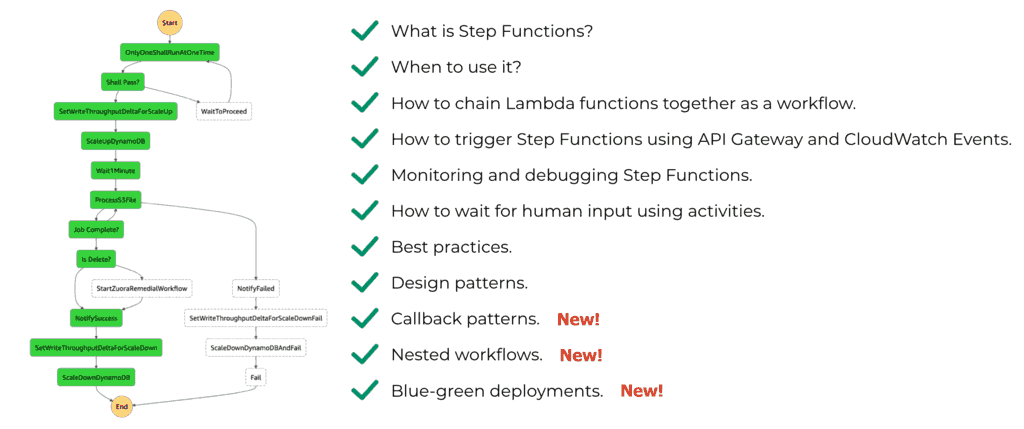# Project Euler – Problem 50 Solution

#### Problem

The prime 41, can be written as the sum of six consecutive primes:

41 = 2 + 3 + 5 + 7 + 11 + 13

This is the longest sum of consecutive primes that adds to a prime below one-hundred.

The longest sum of consecutive primes below one-thousand that adds to a prime, contains 21 terms, and is equal to 953.

Which prime, below one-million, can be written as the sum of the most consecutive primes?

#### Solution

```// generate all prime numbers under <= this max
let max = 10000

// initialise the list with 2 which is the only even number in the sequence

// only check the prime numbers which are <= the square root of the number n
let hasDivisor n =
|> Seq.takeWhile (fun n' -> n' <= int(sqrt(double(n))))
|> Seq.exists (fun n' -> n % n' = 0)

// only check odd numbers <= max
let potentialPrimes = Seq.unfold (fun n -> if n > max then None else Some(n, n+2)) 3

// populate the prime numbers list
for n in potentialPrimes do
if not(hasDivisor n) then primeNumbers  Seq.filter (fun n' -> n' > n)
|> Seq.scan (fun (sum, count) n' -> (sum+n', count+1)) (n, 1)
|> Seq.takeWhile (fun (sum, count) -> sum < 1000000)
|> Seq.filter (fun (sum, count) -> isPrime sum)
|> Seq.maxBy (fun (sum, count) -> count)

// for all numbers, find the longest sequence
let answer = primeNumbers |> Seq.map getPrimeSequence |> Seq.maxBy (fun (sum, count) -> count)
```

Again, utilizing the PGsimple 3 algorithm from here, this solution runs in just over a second as I’ve restricted it to check sequences starting with a prime < 10000 which perhaps unsurprisingly, has provided me with the answer.

The only tricky part in this problem is how to build up a list of sequences for each starting prime and find the longest sequence starting from that number which results in a prime < 1000000. Fortunately you can do this rather easily with Seq.scan in F# which works similarly to Seq.fold but gives you all the intermediate results as well as the final result. Used in conjunction with Seq.takeWhile it allows me to get all the sequences which sums up to a number less than 1000000. The rest is pretty straight forward, filter out the sums that aren’t prime numbers and find the longest sequence, and do this for all the primes we have generated to find the answer to the problem.Hi, I’m Yan. I’m an AWS Serverless Hero and the author of Production-Ready Serverless.

I specialise in rapidly transitioning teams to serverless and building production-ready services on AWS.

Are you struggling with serverless or need guidance on best practices? Do you want someone to review your architecture and help you avoid costly mistakes down the line? Whatever the case, I’m here to help.Check out my new course, Complete Guide to AWS Step Functions. In this course, we’ll cover everything you need to know to use AWS Step Functions service effectively. Including basic concepts, HTTP and event triggers, activities, callbacks, nested workflows, design patterns and best practices.